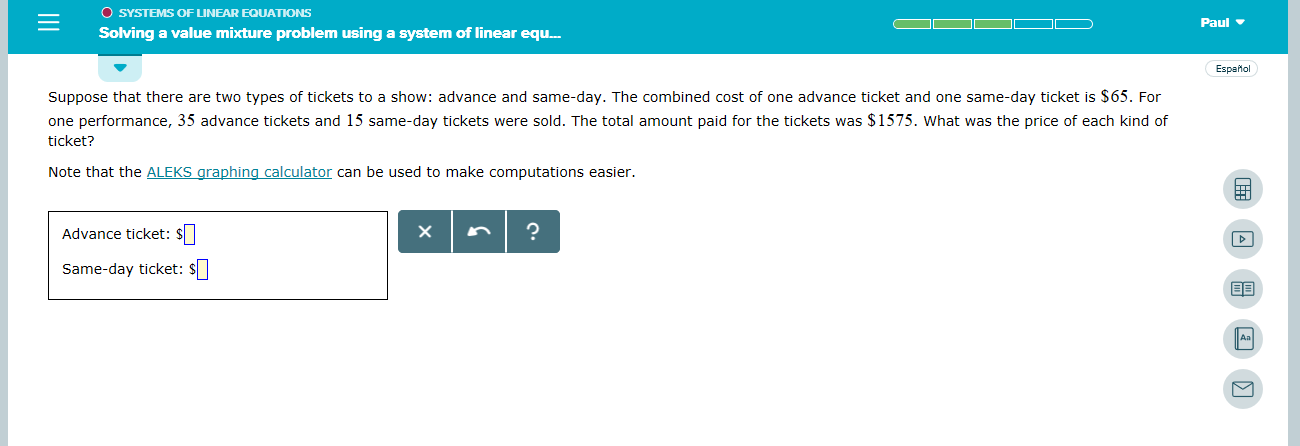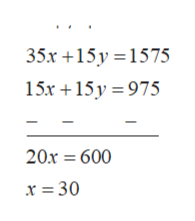O SYSTEMS OF LINEAR EQUATIONSPaulSolving a value mixture problem using a system of linear equ.EspaMolSuppose that there are two types of tickets to a show: advance and same-day. The combined cost of one advance ticket and one same-day ticket is \$65. Forone performance, 35 advance tickets and 15 same-day tickets were sold. The total amount paid for the tickets was \$1575. What was the price of each kind ofticket?Note that the ALEKS graphing calculator can be used to make computations easier.Advance ticket: \$Same-day ticket: S ||Aa

Questionhelp_outlineImage TranscriptioncloseO SYSTEMS OF LINEAR EQUATIONS Paul Solving a value mixture problem using a system of linear equ. EspaMol Suppose that there are two types of tickets to a show: advance and same-day. The combined cost of one advance ticket and one same-day ticket is \$65. For one performance, 35 advance tickets and 15 same-day tickets were sold. The total amount paid for the tickets was \$1575. What was the price of each kind of ticket? Note that the ALEKS graphing calculator can be used to make computations easier. Advance ticket: \$ Same-day ticket: S || Aa fullscreen
Step 1

Let the cost of advance ticket be \$x.

Let the cost  of same day be \$y.

The combined cost of one advanced ticket and one same day ticket is \$65.

Step 2

For one performance, 35 advance ticket and 15 same day ticket were sold and the total cost is 1575, then,

Step 3

Multiply equation 2 with 15 and su...help_outlineImage Transcriptionclose35х +15у%3D1575 15х +15у %3D975 20х 3 600 х %3 30 fullscreen

Want to see the full answer?

See Solution

Want to see this answer and more?

Our solutions are written by experts, many with advanced degrees, and available 24/7

See Solution
Tagged in

Numbers